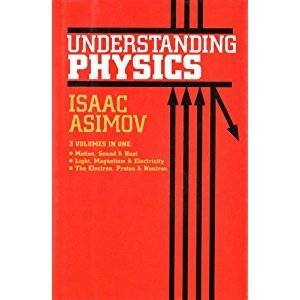# Physics Books

Hi everyone , can you find some books for classical physics for begginers pls ? thnx

Im in first year on high school , i understand math well , so what can you suggest me ?

Im in first year on high school , i understand math well , so what can you suggest me ?Im in first year on high school , i understand math well , so what can you suggest me ?

Could you actually say what mathematics you know please.

How about Conceptual Physics by Hewitt?

How about Conceptual Physics by Hewitt?

thnx you :)thank you Darverz

Could you actually say what mathematics you know please.

well arithmetics ,geometry , algebra , thats all :(

well arithmetics ,geometry , algebra , thats all :(

That should be all you need to get something out of the Conceptual Physics book (probably the Asimov book as well). This is fine, I actually think you will absorb harder books better later on if you get yourself a good basic understanding of how physics works, without having to worry to much about the math.

Once you learn some trigonometry, then "College Physics" by Serway would do you well. The Feynman Lectures also work very well, but I do believe that requires some elementary calculus.

That should be all you need to get something out of the Conceptual Physics book (probably the Asimov book as well). This is fine, I actually think you will absorb harder books better later on if you get yourself a good basic understanding of how physics works, without having to worry to much about the math.

Once you learn some trigonometry, then "College Physics" by Serway would do you well. The Feynman Lectures also work very well, but I do believe that requires some elementary calculus.[/QUOTE

First thank youu , for the books you suggests me.
Can you tell me what calculus need to learn for study physics ?thank you

Generally you need all the basics of calculus for some intro physics course in university..
Usually those calculus-based physics textbooks will give some explanations on math needed..

For calculus-bases physics, I have used 2 text:

-Physics for Scientist and Engineers by Serway/Jewett: it got nice problems. but personally I do not like the explanations

-Fundamentals of Physics by HRW: better explanations, sometimes can be a bit wordy

My advice, if you are still in High School, try to get your fundamentals correct, especially math

Generally you need all the basics of calculus for some intro physics course in university..
Usually those calculus-based physics textbooks will give some explanations on math needed..

For calculus-bases physics, I have used 2 text:

-Physics for Scientist and Engineers by Serway/Jewett: it got nice problems. but personally I do not like the explanations

-Fundamentals of Physics by HRW: better explanations, sometimes can be a bit wordy

My advice, if you are still in High School, try to get your fundamentals correct, especially math

Thank you , i will try